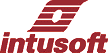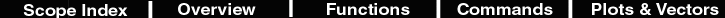Pulse <Width>Pct
 Description Make a pulse 110,20,25,50% of the x axis Makes a new vector which is the length of the default and percent of the span the default vector. The pulse transition will take place at the nearest data point so that quantizing error may be an issue. Hot Key none Script _width = default[length(default)-1] _width=_width - default _width=_width * (.1,.2,.25,.5) result = pulse(_width) plot result Example Open scope and paste this script into the command window. Then press to plot a sine wave Select "Build/pules/Pulse 50Pct, then multiply the result * y and plot it. (result = result * y plot result) set units = rad pi =4*atan(1) numpoints = 1000 time = (vector(numpoints)/(numpoints-1)*1m) newplot sineplot time setplot sineplot y = sin(2*pi*time*1k) plot y set units = deg